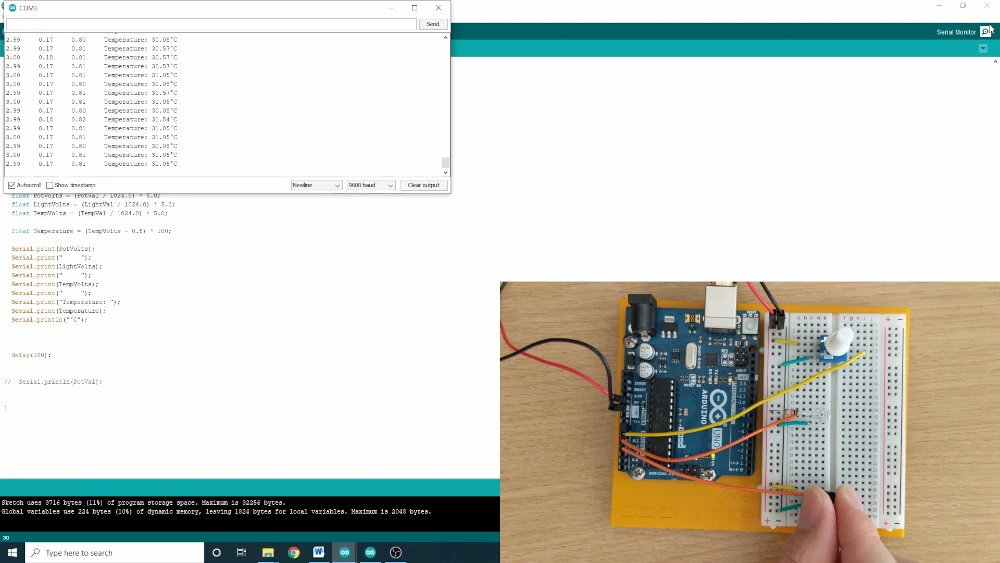Search
•Brandon

# How to Read Analog Sensors with Arduino

Updated: Dec 5, 2020

In this next post of this series, I will demonstrate how to read values from a potentiometer, phototransistor, and temperature sensor using the Arduino analog input pins. In a previous post, I discussed how the digital input pins on the Arduino only read values of HIGH or LOW. With the analog input pins, any value in between 0V and 5V can be read.

Parts Needed

For this exercise you'll need:

• an Arduino Uno,

• potentiometer,

• phototransistor,

• 10kΩ resistor,

• temperature sensor,

• and solid core jumper wires.

These are all included in the Arduino starter kit.

Building the Circuit

This circuit will be used to demonstrate how to read changing values from a potentiometer, phototransistor, and temperature sensor. The potentiometer changes its output voltage when the knob is turned. When the phototransistor is wired in series with a resistor, shown below, it is known as a voltage divider. The measured voltage at the node where the resistor joins the anode of the phototransistor changes based on the amount of light hitting the sensor. The temperature sensor output voltage changes based on the temperature.

• Start by wiring 5V and ground to the power bus rails on the breadboard.

• Wire 5V and ground to the outer leads of the potentiometer.

• Connect analog pin A0 to the center pin of the potentiometer.

• Connect a 10kΩ resistor from 5V to the anode of the phototransistor.

• Then ground the cathode of the phototransistor.

• Connect A1 to the node between the resistor and the potentiometer.

• Next place the temperature sensor in the breadboard with the rounded edge facing away from the Arduino. The pins may need to be bent to fit in the breadboard.

• Connect 5V to the top pin to the temperature sensor.

• Ground the bottom pin of the temperature sensor.

• Connect the center pint to A2Arduino Code

The goal of this sketch is to simply to read and interpret the change that the potentiometer, phototransistor, and temperature sensor cause, using the analog input pins on the Arduino. The Arduino sketch can be found here.

• First define three constant integer values for the three sensors and set them equal to three different analog input pins.

```const int PotPin = A0;
const int LightPin = A1;
const int TempPin = A2;```
• Next, in the setup, set the baud rate to 9600 to enable the serial monitor.

`Serial.begin(9600);`
• In the loop, create integer variables that will represent the raw value that is being read from the three assigned analog pins.

• The "analogRead()" command is used to read voltage values from these pins. This command will output values between 0 and 1023. The value of 0 is for 0 volts and the value of 1023 is for 5 volts.

```int PotVal = analogRead(PotPin);
• Two convert these three variables to a voltage, divide them by 1024.0 and then multiply by 5.0.

• These variables are floating point numbers. It's important to have the ".0" after 1024 and 5 in the calculation.

```float PotVolts = (PotVal / 1024.0) * 5.0;
float LightVolts = (LightVal / 1024.0) * 5.0;
float TempVolts = (TempVal / 1024.0) * 5.0;```
• To go even further and convert the voltage measurement from the temperature sensor, the Arduino Project Book from the starter kit recommends subtracting the voltage by 0.5 and multiplying that difference by 100.

• This will output a temperature in Celsius, and the variable should be a floating point number.

`float Temperature = (TempVolts - 0.5) * 100;`
• Finally, print the values in way that is easiest for you to read. I printed all three voltages and the temperature in Celsius.

```Serial.print(PotVolts);
Serial.print("     ");
Serial.print(LightVolts);
Serial.print("     ");
Serial.print(TempVolts);
Serial.print("     ");
Serial.print("Temperature: ");
Serial.print(Temperature);
Serial.println("°C");```

After the upload, you should that the voltage for A0 changes when you turn the knob on the potentiometer. The voltage being read at A1 changes when you change the lighting conditions. The easiest way to change it is by blocking the light with your finger. An lastly, you can change the voltage being read at A2 by using your finger to change the temperature of the temperature sensor.Reading a Voltage from a PotentiometerReading a Voltage from a PhototransistorMeasuring Temperature with Arduino

Thanks for following along. If you would like to stay up to date with this Introduction to Arduino Series, please subscribe below.

The Bored Robot LLC is a participant in the Amazon Services LLC Associates Program, an affiliate advertising program designed to provide a means for sites to earn advertising fees by advertising and linking to Amazon.com.